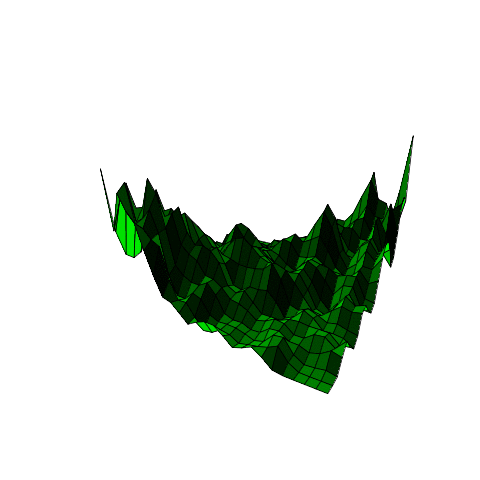# Beta kernel and transformed kernel

This Thursday I will give a talk at Laval University, on “Beta kernel and transformed kernel : applications to copula density estimation and quantile estimation“. This time, I will talk at the department of Mathematics and Statistics (13:30 at the pavillon Adrien-Pouliot). “Because copulas have bounded support (the unit square in dimension 2), standard kernel based estimators of densities are (multiplicatively) biased on borders and in corners of the support. Two techniques can be used to avoid that underestimation: Beta kernels and Transformed kernel. We will describe and discuss those two techniques in the first part of the talk. Then, we will see that it is possible to combine those two techniques to get nice estimator of several quantities (e.g. quantiles): transform the data to get on the unit interval – using a transformed kernel – then estimate the (transformed) quantile on [0,1] using a beta kernel, then get back on the initial support. As we will see on simulations, that technique can be better than standard quantile estimators, especially when data are heavy tailed.” Slides can be downloaded here.• kernel based density estimation

Kernel based estimation are a popular (and natural) technique to estimate densities.  It is simply and extension of the moving histogram:so we count how many observations are a the neighborhood of the point where we want to estimate the density of the distribution. Then it is natural so consider a smoothing function, i.e. instead of a step function (either observations are close enough, or not), it is possible to give weights to observations, which will be a decreasing function of the distance,With a smooth kernel, we have a smooth estimation of the densityThen it is possible to play on the bandwidth, either to get a more accurate estimation of the density, but not that smooth (small bias but large variance),or a smoother one (large bias, but small variance),In R, it is simply

```> X=rnorm(100)
> (D=density(X))

Call:
density.default(x = X)

Data: X (100 obs.);	Bandwidth 'bw' = 0.3548

x                   y
Min.   :-3.910799   Min.   :0.0001265
1st Qu.:-1.959098   1st Qu.:0.0108900
Median :-0.007397   Median :0.0513358
Mean   :-0.007397   Mean   :0.1279645
3rd Qu.: 1.944303   3rd Qu.:0.2641952
Max.   : 3.896004   Max.   :0.3828215

> plot(D\$x,D\$y)```
• Beta kernel

The idea of Beta kernel is to consider kernels having support [0,1]. In the univariate case,whereis the density of a Beta distribution, i.e.For additional material, I have uploaded some R code to fit copula densities using beta kernels,

```library(copula)
beta.kernel.copula.surface = function (u,v,bx,by,p) {
s = seq(1/p, len=(p-1), by=1/p)
mat = matrix(0,nrow = p-1, ncol = p-1)
for (i in 1:(p-1)) {
a = s[i]
for (j in 1:(p-1)) {
b = s[j]
mat[i,j] = sum(dbeta(a,u/bx,(1-u)/bx) *
dbeta(b,v/by,(1-v)/by)) / length(u)
} }
return(data.matrix(mat)) }```

Then we can used it to see what we get on a simulated sample

```library(copula)
COPULA = frankCopula(param=5, dim = 2)
X = rcopula(n=1000,COPULA)
p0 = 26
Z= beta.kernel.copula.surface(X[,1],X[,2],bx=.01,by=.01,p=p0)
u = seq(1/p0, len=(p0-1), by=1/p0)
box=FALSE,zlim=c(0,6))```(yes, the surface is changing… to illustrate the impact of the bandwidth on the estimation).

• transformed kernel estimationI the talk, I will also mention the transformed Kernel estimate, as introduced in the book on L1 density estimation by Luc Devroye and Laszlo Györfi (the book can be downloaded here). I probably spend a few minutes on the original chapter, in order to provide another application of that techniques (not only to estimate copula densities, but here to estimate quantiles of heavy tailed distribution). In the univariate case, the R code is the following (here I consider two transformation, the quantile function of the Gaussian distribution, and the quantile function of the Student distribution with 3 degrees of freedom),

```set.seed(1)
sample=rbeta(100,4,3)

transfN = function(x){
Y=qnorm(sample)
f=density(Y,from=-4,to=4,n=2001)
ny=sum(f\$x<=qnorm(x));
g=f\$y[ny]/dnorm(qnorm(x))
return(g)
}

df0=3

transfT = function(x){
Y=qt(sample,df=df0)
f=density(Y,from=-4,to=4,n=2001)
ny=sum(f\$x<=qt(x,3));
g=f\$y[ny]/dt(qt(x,df=df0),df=df0)
return(g)
}

tN=Vectorize(transfN)
tT=Vectorize(transfT)

u=seq(.01,.99,by=.01)
vN=tN(u)
vT=tT(u)
plot(u,vN,type="l",lwd=3,col="blue")
lines(u,vT,lwd=3,col="green")
lines(u,dbeta(u,4,3),col="red",lty=2)```

The density estimation is the following,(the red dotted line is the true density, since we work on a simulated sample). Now, let us get back on the initial chapter,In the book, this is introduced as follows,The original idea we add it to use this kernel based estimator for copulas, i.e. since we can estimate densities in high dimension with unbounded support, usingthe idea is to transform marginal observations,and to use the fact that the associated copula density can be writtento derive an intuitive estimator for the copula densityAn important issue is how do we choose the transformationAnd Luc Devroye and Laszlo Györfi mention that this can be used to deal with extremes.well, extremes are introduced through bumps (which is not the way I would have been dealing with extremes)and note that several results can be derived on those bumps,e.g.Then, there is an interesting discussion about estimating the optimal transformationand I will prove that this can be an extremely interesting idea, for instance to estimate quantiles of heavy tailed distribution, if we use also the beta kernel estimator on the unit interval. This idea was developed in a paper with Abder Oulidi, online here.

Remark: actually, in the book, an additional reference is mentioned,but I have never been able to find a copy… if anyone has one, I’d be glad to read it…

## One thought on “Beta kernel and transformed kernel”

1.javad says:

i need Parkinson,
Haberman’s survival, Satellite datasets run with r.

This site uses Akismet to reduce spam. Learn how your comment data is processed.